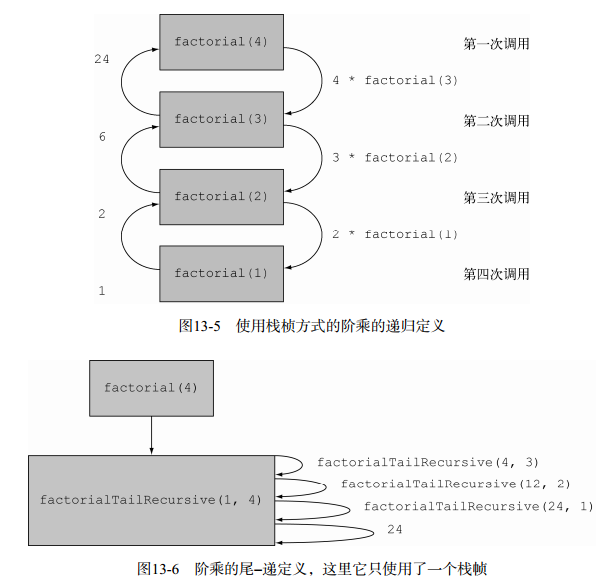# Java8 In Action-3.超越 Java 8 (一)

1.函数式的思考
1.1实现和维护系统

 除了构造器内的初始化操作，对类中数据结构的任何修改，包括字段的赋值操作（一个典型的例子是setter方法）。
 抛出一个异常。
 进行输入/输出操作，比如向一个文件写数据。

1.2什么是函数式编程

“没有可感知的副作用”（不改变对调用者可见的变量、不进行I/O、不抛出异常）的这些限制都隐含着引用透明性。如果一个函数只要传递同样的参数值，总是返回同样的果，那这个函数就是引用透明的。String.replace方法就是引用透明的， 因像"raoul".replace(‘r’,‘R’)这样的调用总是返回同样的结果（replace方法返回一个新的字符串，用小写的r替换掉所有大写的R） ，而不是更新它的this对象，所以它可以被看成函数式的。换句话说，函数无论在何处、何时调用，如果使用同样的输入总能持续地得到相同的结果，就具备了函数式的特征。通常情况下， 在函数式编程中，你应该选择使用引用透明的函数。

package com.h.java8;

import java.util.ArrayList;
import java.util.Arrays;
import java.util.Collections;
import java.util.List;

/**
* Created by John on 2018/9/23.
*/
public class TestMain {

public static void main(String[] args) {
List<Integer> list = Arrays.asList(1,4,9);
List<List<Integer>> subsets = subsets(list);
subsets.forEach(System.out::println);
}

/**
* 求集合的子集
* 基本思想:递归的将集合的第一个元素插入到其他元素构成的子集中
* @param list
* @return
*/
public static List<List<Integer>> subsets(List<Integer> list){
if (list.isEmpty()){
List<List<Integer>> ans = new ArrayList<>();
return ans;
}
Integer first = list.get(0);
List<Integer> rest = list.subList(1,list.size());
List<List<Integer>> subans = subsets(rest);
List<List<Integer>> subans2 = insertAll(first, subans);
return concat(subans, subans2);
}

public static List<List<Integer>> insertAll(Integer first, List<List<Integer>> lists) {
List<List<Integer>> result = new ArrayList<>();
for (List<Integer> list : lists) {
List<Integer> copyList = new ArrayList<>();
}
return result;
}

public static List<List<Integer>> concat(List<List<Integer>> a, List<List<Integer>> b) {
/**
* 常见的版本
* return a;
*/
/**
* 改进后版本的优势:
* 纯粹的函数式,虽然它在内部会对对象进行修改（向列表r添加元素），但是它返回的结果基于参数却没有修改任何一个传入的参数。
* 与此相反，第一个版本基于这样的事实，执行完concat(subans, subans2)方法调用后，没人需要再次使用subans的值。
* 对于我们定义的subsets，这的确是事实，所以使用简化版本的concat是个不错的选择。
* 不过，这也取决于你如何审视你的时间，你是愿意为定位诡异的缺陷费劲心机耗费时间呢？还是花费些许的代价创建一个对象的副本呢？
* 请牢记：考虑编程问题时，采用函数式的方法，关注函数的输入参数以及输出结果（即你希望做什么），
* 通常比设计阶段的早期就考虑如何做、修改哪些东西要卓有成效得多。
*/
List<List<Integer>> r = new ArrayList<>(a);
return r;
}
}



1.3递归和迭代

package com.h.java8;

import java.util.stream.LongStream;

/**
* Created by John on 2018/9/23.
*/
public class TestMain {

public static void main(String[] args) {
long start = System.currentTimeMillis();
//long result = factorialIterative(4);
// long result = factorialRecursive(4);
//long result = factorialStreams(4);
long result = factorialTailRecursive(4);
System.out.println(result);
long end = System.currentTimeMillis();
System.out.println("耗时:" + (end - start) + "ms");
long fibonacci = fibonacci(12);
long fibonacci2 = fibonacciTailRecursive(12);
System.out.println(fibonacci);
System.out.println(fibonacci2);
}

/**
* 迭代式阶乘
* @param n
*/
public static long factorialIterative(long n){
long result = 1;
for (long i=1;i<=n;i++){
result *= i;
}
return result;
}

/**
* 递归式阶乘
* 优点:易于实现和理解
* 缺点:执行效率上不如迭代,且当n很大时,容易发生java.lang.StackOverflowError
* 通常而言，执行一次递归式方法调用的开销要比迭代执行单一机器级的分支指令大不少
* 因为递归中的每次方法调用都会在调用栈上创建一个新的栈帧,用于保存每个方法调用的状态,这个操作直到递归结束,
* 这意味着递归方法会依据它接收的输入成比例的消耗内存.
* @param n
* @return
*/
public static long factorialRecursive(long n){
return  n == 1 ? 1:n*factorialRecursive(n-1);
}

/**
* 基于Stream的阶乘
* @param n
* @return
*/
public static long factorialStreams(long n){
return LongStream.rangeClosed(1,n).reduce(1,(a,b) -> a*b);
}

/**
* 尾递归
* 基本的思想:编写阶乘的一个迭代定义，不过迭代调用发生在函数的最后（所以我们说调用发生在尾部）。
* 这种新型的迭代调用经过优化后执行的速度快很多。(但目前Java还不支持这种优化)
* 优点:不需要在不同的栈帧上保存每次递归调用的中间值,编译器能够自行决定复用某个栈帧进行计算
* 在 factorialHelper(long acc,long n)方法中的立即数(中间结果)直接作为参数传递给了该方法,
* 再也不用为每个递归调用分配单独的栈帧用于跟踪每次递归调用的中间值——通过方法的参数能够直接访问这些值。
* @param n
* @return
*/
public static long factorialTailRecursive(long n){
return factorialHelper(1,n);
}

public static long factorialHelper(long acc,long n){
return n == 1 ? acc:factorialHelper(acc*n,n-1);
}

/**
* 斐波那契数列
* f(n)=1 (n=0,n=1)
* f(n)=f(n-1) + f(n-2) n>=2
* @param n
* @return
*/
public static long fibonacci(long n){
if (n == 0 || n == 1){
return 1;
}
return fibonacci(n-1) + fibonacci(n-2);
}

/**
* 基于尾递归的斐波那契数列
* @param n
* @return
*/
public static long fibonacciTailRecursive(long n){
if (n == 0 || n == 1){
return 1;
}
return fibonacciHelper(1,1,n);
}

public static long fibonacciHelper(long f0,long f1,long n){
return n == 2 ? f0+f1:fibonacciHelper(f1,f0+f1,n-1);
}
}1.4小结
 从长远看，减少共享的可变数据结构能帮助你降低维护和调试程序的代价。
 函数式编程支持无副作用的方法和声明式编程。
 函数式方法可以由它的输入参数及输出结果进行判断。
 如果一个函数使用相同的参数值调用，总是返回相同的结果，那么它是引用透明的。采用递归可以取得迭代式的结构，比如while循环。
 相对于Java语言中传统的递归，“尾递”可能是一种更好的方式，它开启了一扇门，让我们有机会最终使用编译器进行优化。02-12497
09-10503
11-06231
10-04205
04-03217
03-30673
02-1516
11-06165
07-01686
07-1718
05-0786
08-27
03-28410